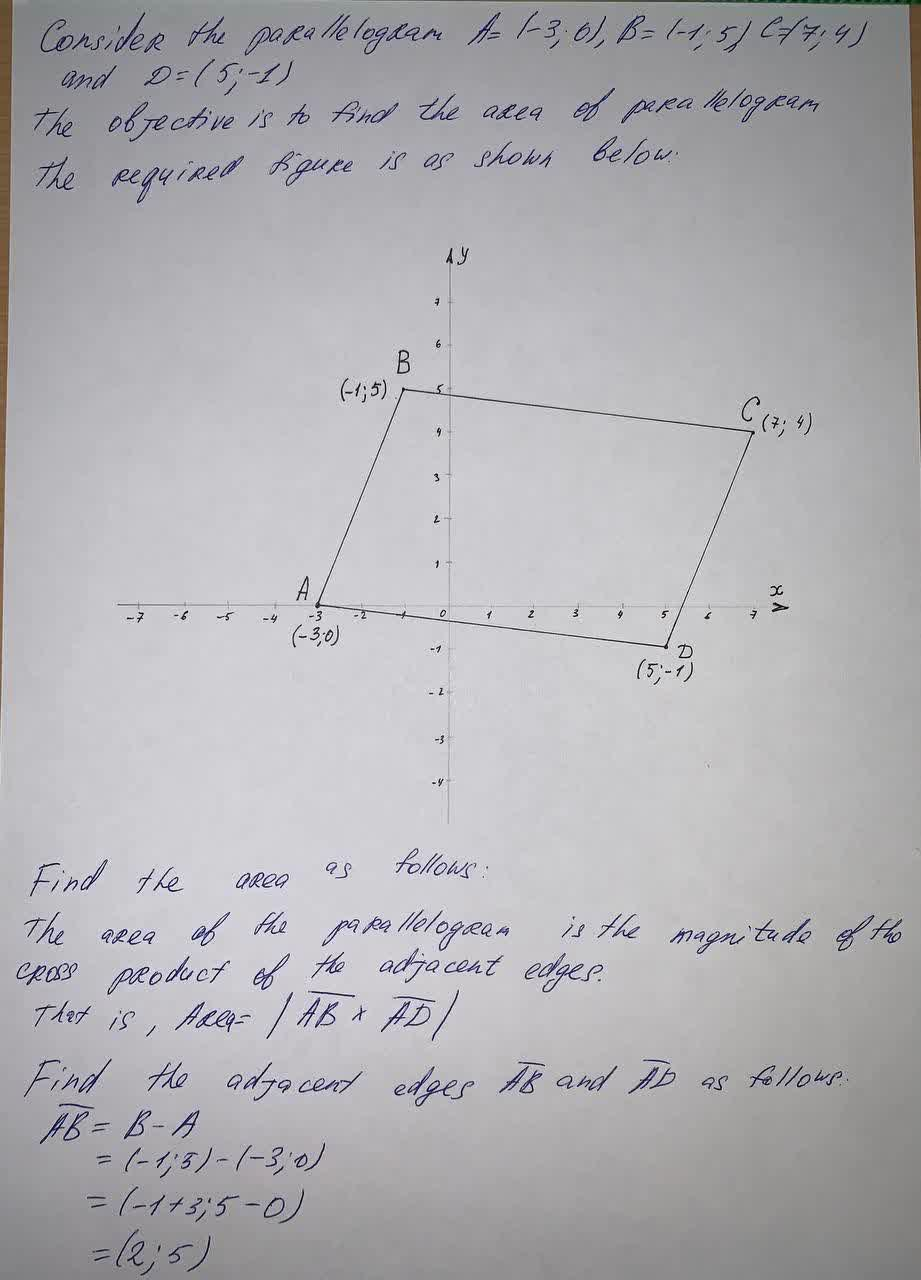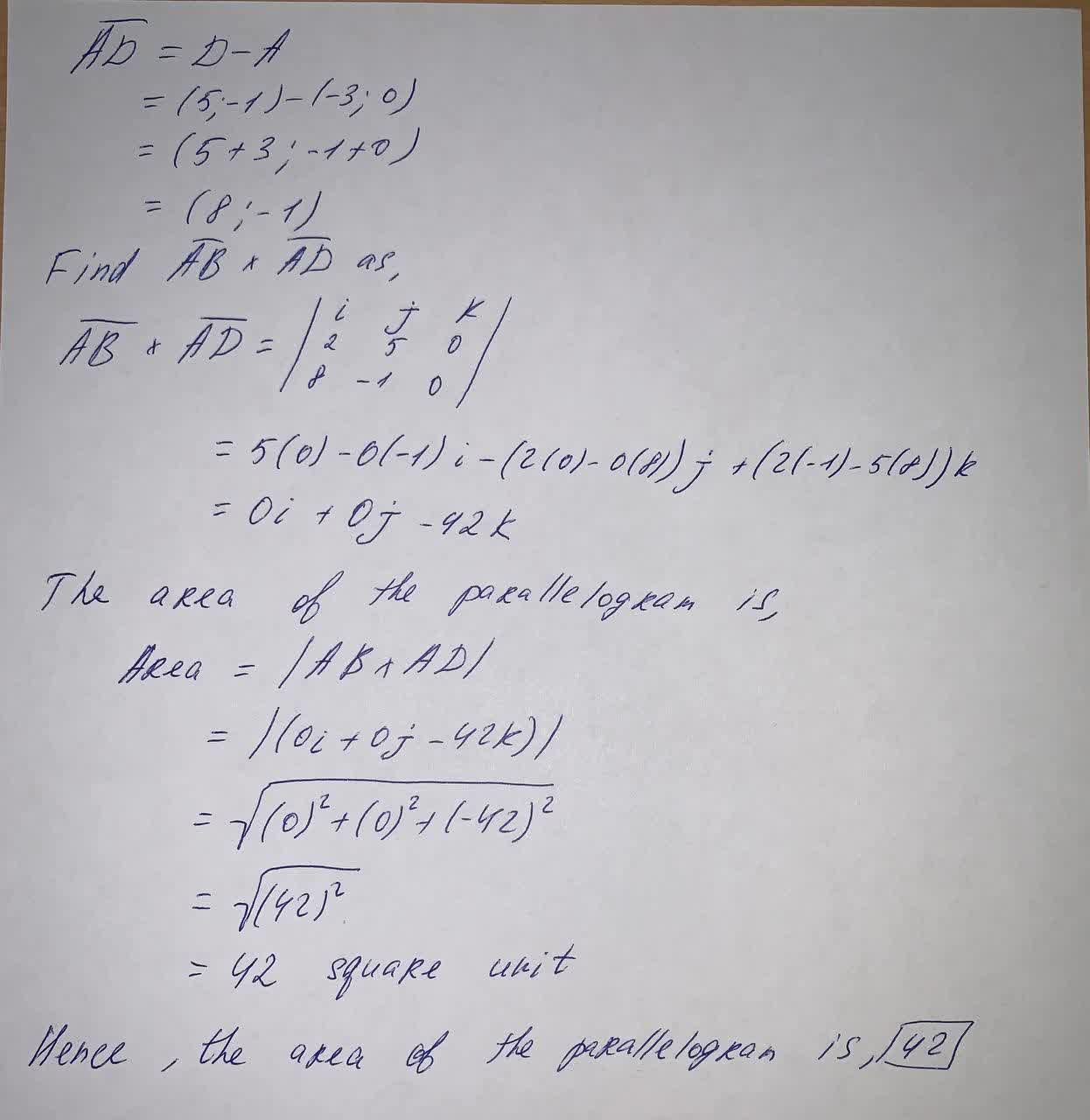# Find the area of the parallelogram with vertices A(-3, 0), B(-1, 5), C(7, 4), and D(5, -1).iohanetc 2021-06-11 Answered
Find the area of the parallelogram with vertices A(-3, 0), B(-1, 5), C(7, 4), and D(5, -1).

• Questions are typically answered in as fast as 30 minutes

### Plainmath recommends

• Get a detailed answer even on the hardest topics.
• Ask an expert for a step-by-step guidance to learn to do it yourself.un4t5o4v###### Have a similar question?content_user

Consider the parallelogram A=(-3;0), B(-1,5), C(7;4) and D=(5;-1)

The objective is to find the area of parallelogram

Find the are as follows:

The area of the parallelogram is the magnitude of the cross product of the adjacent edges.

That is, $$Area=|\overline{AB}\times\overline{AD}|$$

Find the adjacent edges $$\overline{AB}$$ and $$\overline{AD}$$ as follows

$$\overline{AB}=B-A$$

$$=(-1;5)-(-3;0)$$

$$=(-1+3;5-0)$$

$$=(2;5)$$

$$\overline{AD}=D-A$$

$$=(5;-1)-(-3;0)$$

$$=(8;1)$$

Find $$\overline{AB}\times\overline{AD}$$ as,

$$\overline{AB}\times\overline{AD}=\begin{bmatrix}i&j&k\\2&5&0\\8&-1&0\end{bmatrix}$$

$$=5(0)-0(-1)i-(2(0)-0(8))j+(2(-1)-5(8))k$$

$$=0i+0j-42k$$

The area of the parallelogram is,

Area $$=|AB\times AD|$$

$$=|(0i+0j-42k|$$

$$=\sqrt{(0)^2+(0)^2+(-42)^2}$$

$$=\sqrt{(42)^2}$$

= 42 square unit

Hence, the area of parallelogram is 42